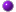-

## Numerology - How To Calculate Your Numbers

How To Calculate Your Own Numbers In Just Minutes.

The process numerologists use to reduce a number or a word is known as digit summing, then make the interpretation based on the single digit that is produced.

Digit summing involves adding up the sum of all of the digits in a number, and repeating the process if necessary until a single-digit answer is produced. For a word, the values corresponding to each letter's place in the alphabet (e.g., A=1, B=2, through Z=26) are summed.

Examples:

---3,489 ---> 3 + 4 + 8 + 9 = 24 -> 2 + 4 = 6

---Hello ---> 8 + 5 + 3 + 3 + 6 = 52 -> 5 + 2 = 7

 1 2 3 4 5 6 7 8 9 A B C D E F G H I J K L M N O P Q R S T U V W X Y Z

Let's use another example with the name John Smith. Following the chart above we get...

J    O   H   N    S    M    I    T   H
1 + 3 + 8 + 5 + 1 + 4 + 9 + 2 + 8 = 41

41 is a double digit so we must add the two numbers until we arrive at a single digit.
In this example the 4 + 1 = 5

The resulting numbers are provide meaning and insight into ones life,personal path and future. The definitions for the meaning of each number varies among different cultures and systems. Frequently, the number 1 usually corresponds to the individual or self. The number 2 is associated with duality and union. Beyond the number 2, definitions may vary.

There are many different methods of calculations include Chaldean, Pythagorean, Hebraic, Helyn Hitchcock's method, Phonetic, Japanese and Indian.

In most cases, numerology uses just the name and birthdate of an individual to analyze and define the personality or forecast.

The following is an excerpt from a numerology page at globalpsychics.com on numerology calculation methods.

CHALDEAN/KABBALAH METHOD is said to be only true and reliable system. It was originated by ancient Chaldeans, passed on to the Hebrews. The knowledge became part of the Kabbalah (Jewish mysticism/mystical tradition). This system of numerological calculation was taught to Jesus by the Essenes.

Western/Pythagorean Method doesnt work as well because the values arent based on sound vibration  the Western system is considered more of a "Parlour Game", partly because it doesnt emphasize compound numbers (destiny).

There is scientific rationale correlating sound vibration with organic patterns.Pythagoras (Greek philosopher & mathematician 2,500 yrs ago) devised a system of numerological calculation that was lost when he fell from favour. Some years later a student of his assigned numbers to the letters of the alphabet, sequentially, instead of according to sound vibration. This system is damaged, as our modern alphabet didnt exist then. Interesting, the Pythagorean system formed the spiritual basis for many secret societies e.g. the Masons.

Visitglobalpsychics.com to learn how to calculate using the Chaldean/Kabbalah method.

For the sake of simplicity, the Dimension1111.com numerology calculations are based on the Pythagoras method and is best for a beginner who just wants to get the feel for how numerology works. If this is a subject that interests you then consider exploring and learning about all the various methods and traditions of Numerology.Next Section: Number MeaningsPrevious PageTop of Page

The time is now on